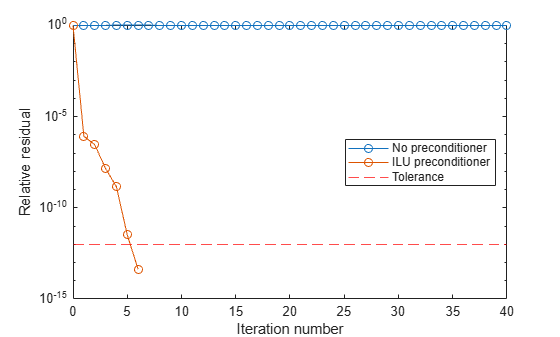Documentation

tfqmr

Transpose-free quasi-minimal residual method

Syntax

x = tfqmr(A,b)
x = tfqmr(afun,b)
x = tfqmr(a,b,tol)
x = tfqmr(a,b,tol,maxit)
x = tfqmr(a,b,tol,maxit,m)
x = tfqmr(a,b,tol,maxit,m1,m2,x0)
[x,flag] = tfqmr(A,B,...)
[x,flag,relres] = tfqmr(A,b,...)
[x,flag,relres,y]y(A,b,...)
[x,flag,relres,iter,resvec] = tfqmr(A,b,...)

Description

x = tfqmr(A,b) attempts to solve the system of linear equations A*x=b for x. The n-by-n coefficient matrix A must be square and the right-hand side column vector b must have length n.

x = tfqmr(afun,b) accepts a function handle, afun, instead of the matrix A. The function, afun(x), accepts a vector input x and returns the matrix-vector product A*x. In all of the following syntaxes, you can replace A by afun. Parameterizing Functions explains how to provide additional parameters to the function afun.

x = tfqmr(a,b,tol) specifies the tolerance of the method. If tol is [] then tfqmr uses the default, 1e-6.

x = tfqmr(a,b,tol,maxit) specifies the maximum number of iterations. If maxit is [] then tfqmr uses the default, min(N,20).

x = tfqmr(a,b,tol,maxit,m) and x = tfqmr(a,b,tol,maxit,m1,m2) use preconditioners m or m=m1*m2 and effectively solve the system A*inv(M)*x = B for x. If M is [] then a preconditioner is not applied. M may be a function handle mfun such that mfun(x) returns m\x.

x = tfqmr(a,b,tol,maxit,m1,m2,x0) specifies the initial guess. If x0 is [] then tfqmr uses the default, an all zero vector.

[x,flag] = tfqmr(A,B,...) also returns a convergence flag:

Flag

Convergence

0

tfqmr converged to the desired tolerance tol within maxit iterations.

1

tfqmr iterated maxit times but did not converge.

2

Preconditioner m was ill-conditioned.

3

tfqmr stagnated. (Two consecutive iterates were the same.)

4

One of the scalar quantities calculated during tfqmr became too small or too large to continue computing.

[x,flag,relres] = tfqmr(A,b,...) also returns the relative residual norm(b-A*x)/norm(b). If flag is 0, then relres <= tol.

[x,flag,relres,y]y(A,b,...) also returns the iteration number at which x was computed: 0 <= iter <= maxit.

[x,flag,relres,iter,resvec] = tfqmr(A,b,...) also returns a vector of the residual norms at each iteration, including norm(b-A*x0).

Examples

Using tfqmr with Matrix or Function Handle Input

This example shows how to use tfqmr with a matrix input and with a function input.

n = 100; on = ones(n,1);
A = spdiags([-2*on 4*on -on],-1:1,n,n);
b = sum(A,2);
tol = 1e-8;
maxit = 15;
M1 = spdiags([on/(-2) on],-1:0,n,n);
M2 = spdiags([4*on -on],0:1,n,n);
x = tfqmr(A,b,tol,maxit,M1,M2,[]);

You can also use a matrix-vector product function as input:

function y = afun(x,n)
y = 4 * x;
y(2:n) = y(2:n) - 2 * x(1:n-1);
y(1:n-1) = y(1:n-1) - x(2:n);
x1 = tfqmr(@(x)afun(x,n),b,tol,maxit,M1,M2);

If applyOp is a function suitable for use with qmr, it may be used with tfqmr by wrapping it in an anonymous function:

x1 = tfqmr(@(x)applyOp(x,'notransp'),b,tol,maxit,M1,M2);

Using tfqmr with a Preconditioner

This example demonstrates the use of a preconditioner.

Load A = west0479, a real 479-by-479 nonsymmetric sparse matrix.

A = west0479;

Define b so that the true solution is a vector of all ones.

b = full(sum(A,2));

Set the tolerance and maximum number of iterations.

tol = 1e-12;
maxit = 20;

Use tfqmr to find a solution at the requested tolerance and number of iterations.

[x0,fl0,rr0,it0,rv0] = tfqmr(A,b,tol,maxit);

fl0 is 1 because tfqmr does not converge to the requested tolerance 1e-12 within the requested 20 iterations. The seventeenth iterate is the best approximate solution and is the one returned as indicated by it0 = 17. MATLAB® stores the residual history in rv0.

Plot the behavior of tfqmr.

semilogy(0:maxit,rv0(1:maxit+1)/norm(b),'-o');
xlabel('Iteration number');
ylabel('Relative residual');Note that like bicgstab, tfqmr keeps track of half iterations. The plot shows that the solution does not converge. You can use a preconditioner to improve the outcome.

Create the preconditioner with ilu, since the matrix A is nonsymmetric.

[L,U] = ilu(A,struct('type','ilutp','droptol',1e-5));
Error using ilu
There is a pivot equal to zero. Consider decreasing
the drop tolerance or consider using the 'udiag' option.

MATLAB cannot construct the incomplete LU as it would result in a singular factor, which is useless as a preconditioner.

You can try again with a reduced drop tolerance, as indicated by the error message.

[L,U] = ilu(A,struct('type','ilutp','droptol',1e-6));
[x1,fl1,rr1,it1,rv1] = tfqmr(A,b,tol,maxit,L,U);

fl1 is 0 because tfqmr drives the relative residual to 4.1410e-014 (the value of rr1). The relative residual is less than the prescribed tolerance of 1e-12 at the sixth iteration (the value of it1) when preconditioned by the incomplete LU factorization with a drop tolerance of 1e-6. The output rv1(1) is norm(b), and the output rv1(7) is norm(b-A*x2).

You can follow the progress of tfqmr by plotting the relative residuals at each iteration starting from the initial estimate (iterate number 0).

semilogy(0:0.5:it1,rv1/norm(b),'-o');
xlabel('Iteration number');
ylabel('Relative residual');